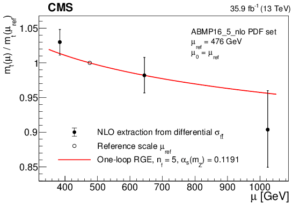## CMS catches the top quark running

CMS catches the top quark running

Article : “Running of the top quark mass from proton-proton collisions at √ s = 13 TeV“

Authors: The CMS Collaboration

Reference: https://arxiv.org/abs/1909.09193

When theorists were first developing quantum field theory in the 1940’s they quickly ran into a problem. Some of their calculations kept producing infinities which didn’t make physical sense. After scratching their heads for a while they eventually came up with a procedure known as renormalization to solve the problem.  Renormalization neatly hid away the infinities that were plaguing their calculations by absorbing them into the constants (like masses and couplings) in the theory, but it also produced some surprising predictions. Renormalization said that all these ‘constants’ weren’t actually constant at all! The value of these ‘constants’ depended on the energy scale at which you probed the theory.

One of the most famous realizations of this phenomena is the ‘running’ of the strong coupling constant. The value of a coupling encodes the strength of a force. The strong nuclear force, responsible for holding protons and neutrons together, is actually so strong at low energies our normal techniques for calculation don’t work. But in 1973, Gross, Wilczek and Politzer realized that in quantum chromodynamics (QCD), the quantum field theory describing the strong force, renormalization would make the strong coupling constant ‘run’ smaller at high energies. This meant at higher energies one could use normal perturbative techniques to do calculations. This behavior of the strong force is called ‘asymptotic freedom’ and earned them a Nobel prize. Thanks to asymptotic freedom, it is actually much easier for us to understand what QCD predicts for high energy LHC collisions than for the properties of bound states like the proton.Figure 1: The value of the strong coupling constant (α_s) is plotted as a function of the energy scale. Data from multiple experiments at different energies are compared to the prediction from QCD of how it should run.  From Now for the first time, CMS has measured the running of a new fundamental parameter, the mass of the top quark. More than just being a cool thing to see, measuring how the top quark mass runs tests our understanding of QCD and can also be sensitive to physics beyond the Standard Model. The top quark is the heaviest fundamental particle we know about, and many think that it has a key role to play in solving some puzzles of the Standard Model. In order to measure the top quark mass at different energies, CMS used the fact that the rate of producing a top quark-antiquark pair depends on the mass of the top quark. So by measuring this rate at different energies they can extract the top quark mass at different scales.

Top quarks nearly always decay into W-bosons and b quarks. Like all quarks, the b quarks then create a large shower of particles before they reach the detector called a jet. The W-bosons can decay either into a lepton and a neutrino or two quarks. The CMS detector is very good at reconstructing leptons and jets, but neutrinos escape undetected. However one can infer the presence of neutrinos in an event because we know energy must be conserved in the collision, so if neutrinos are produced we will see ‘missing’ energy in the event. The CMS analyzers looked for top anti-top pairs where one W-boson decayed to an electron and a neutrino and the other decayed to a muon and a neutrino. By using information about the electron, muon, missing energy, and jets in an event, the kinematics of the top and anti-top pair can be reconstructed.

The measured running of the top quark mass is shown in Figure 2. The data agree with the predicted running from QCD at the level of 1.1 sigma, and the no-running hypothesis is excluded at above 95% confidence level. Rather than being limited by the amount of data, the main uncertainties in this result come from the theoretical understanding of the top quark production and decay, which the analyzers need to model very precisely in order to extract the top quark mass. So CMS will need some help from theorists if they want to improve this result in the future.Figure 2: The ratio of the top quark mass compared to its mass at a reference scale (476 GeV) is plotted as a function of energy. The red line is the theoretical prediction of how the mass should run in QCD.